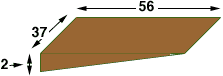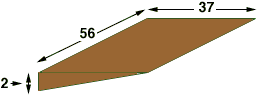Quandaries and Queries Name: joe Who is asking: Other Level: All Question: We just bought a house and need to start filling in the back yard with dirt to level it out. I measure 37'x 56' by 2' deep on an angle. I will be building a retaining wall at the 2' deep portion. The slop will go from the 2' to nothing at the top or near the house. I can't remember how to get the Sq. foot or cubic yards. Hi Joe, From your description I see the "wedge" of dirt you need as in one of the diagrams below.If one of these diagrams is correct then the volume is the length times the width times half the depth. In either case this would be 37561 = 2072 cubic feet. If you need it in cubic yards then express each of the dimensions in yards before you calculate the volume. There are 3 feet in a yard so divide each of the dimessions by 3 to convert to yards. The volume is then (37/3)(56/3)(1/3) = 76.7 cubic yards. Penny Go to Math Central